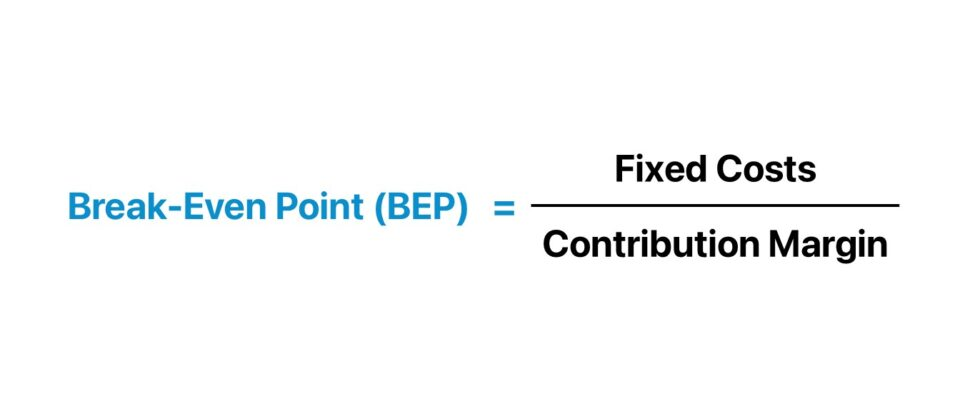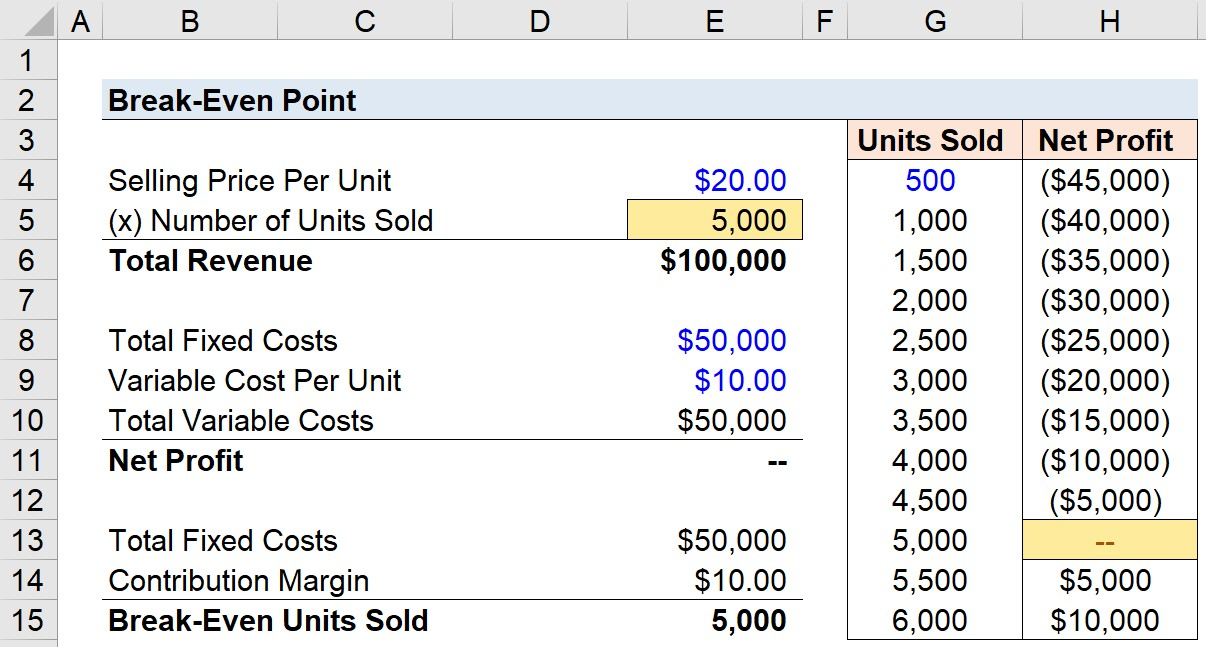Welcome to Wall Street Prep! Use code at checkout for 15% off.# Break Even Point (BEP)

Guide to Understanding the Break Even Point (BEP)## How to Calculate Break-Even Point?

There is no net loss or gain at the break-even point (BEP), but the company is now operating at a profit from that point onward.

For all business owners, particularly during the earlier stages of a business, one of the most crucial questions to answer is: “When will my business break even?”

Businesses share the similar core objective of eventually becoming profitable in order to continue operating. Otherwise, the business will need to wind-down since the current business model is not sustainable.

An unprofitable business eventually runs out of cash on hand, and its operations can no longer be sustained (e.g., compensating employees, purchasing inventory, paying office rent on time).

By understanding the required output to break even, a company can set revenue targets accordingly, as well as adjust its business strategy such as the pricing of its products/services and how it chooses to allocate its capital.

If a company has reached its break-even point, this means the company is operating at neither a net loss nor a net gain (i.e. “broken even”).

The incremental revenue beyond the break-even point (BEP) contributes toward the accumulation of more profits for the company.

Conducting a break-even analysis is a prerequisite to setting prices appropriately, establishing clear and logical sales target goals, and identifying weaknesses in the current state of the business model that could benefit from improvements (e.g., sales tactics and marketing strategies).

Furthermore, established companies with a diverse portfolio of product/service offerings can estimate the break-even point on an individualized product-level basis to assess whether adding a certain product would be economically viable. In effect, the analysis enables setting more concrete sales goals as you have a specific number to target in mind.

## Break-Even Point Formula (BEP)

The formula for calculating the break-even point (BEP) involves taking the total fixed costs and dividing the amount by the contribution margin per unit.

Break-Even Point (BEP) = Fixed Costs ÷ Contribution Margin

To take a step back, the contribution margin is the selling price per unit minus the variable costs per unit, and represents the amount of revenue remaining after meeting all the associated variable costs accumulated to generate that revenue.

• Contribution Margin = Fixed Costs→ That said, when a company’s contribution margin (in dollar terms) is equal to its fixed costs, the company is at its break-even point.
• Contribution Margin > Fixed Costs→ If the company’s contribution margin exceeds its fixed costs, then the company actually starts profiting from the sale of its products or services.

## Quick Break Even Analysis Example (BEP)

For example, if a company has \$10,000 in fixed costs per month, and their product has an average selling price (ASP) of \$100, and the variable cost is \$20 for each product, that comes out to a contribution margin per unit of \$80.

• Fixed Costs per Month = \$10,000
• Average Selling Price (ASP) = \$100.00
• Variable Cost per Unit = \$20.00
• Contribution Margin = \$80.00

Then, by dividing \$10k in fixed costs by the \$80 contribution margin, you’ll end up with 125 units as the break-even point, meaning that if the company sells 125 units of its product, it’ll have made \$0 in net profit.

• Break-Even Point (BEP) = 125 Units

Or, if using Excel, the break-even point can be calculated using the “Goal Seek” function.

After entering the end result being solved for (i.e., the net profit of zero), the tool determines the value of the variable (i.e., the number of units that must be sold) that makes the equation true.

## Break-Even Point Calculator (BEP)

We’ll now move to a modeling exercise, which you can access by filling out the form below.Submitting...

## 1. Unit Economics and Cost Structure Assumptions

Let’s say that we have a company that sells products priced at \$20.00 per unit, so revenue will be equal to the number of units sold multiplied by the \$20.00 price tag.

• Selling Price Per Unit = \$20.00

In terms of its cost structure, the company has fixed costs (i.e., constant regardless of production volume) that amounts to \$50k per year. Recall, fixed costs are independent of the sales volume for the given period, and include costs such as the monthly rent, the base employee salaries, and insurance.

• Total Fixed Costs = \$50,000

Moving onto our final assumption, the variable costs directly associated with the production of the products being sold are \$10.00.

• Variable Costs = \$10.00 Per Unit

In contrast to fixed costs, variable costs increase (or decrease) based on the number of units sold. If customer demand and sales are higher for the company in a certain period, its variable costs will also move in the same direction and increase (and vice versa).

The total variable costs will therefore be equal to the variable cost per unit of \$10.00 multiplied by the number of units sold.

## 2. Excel Goal Seek Function

We now have the assumptions in place to use the “Goal Seek” function in Excel (Alt ➝ A ➝ W ➝ G).

• Step 1 → First, we link to the net profit cell for the “Set cell” selection
• Step 2 → In the subsequent step, we are going to input zero as the “To value” since the profit we are targeting is \$0 (i.e., the break-even point)
• Step 3 → Lastly, the “By changing cell” will be set to the number of units sold, as this is the variable that Excel will be adjusting until our target profit value is met

Upon doing so, the number of units sold cell changes to 5,000, and our net profit is equal to zero, as shown below in the screenshot of the finished solution.

## 3. Break-Even Point Analysis Example

Quantifying the required number of units to be sold to have a net profit of zero was our intended goal, which in this particular scenario, the company must sell 5k units of its products to break even and start to generate a profit from its operations.

As we can see from the sensitivity table, the company operates at a loss until it begins to sell products in quantities in excess of 5k. For instance, if the company sells 5.5k products, its net profit is \$5k.

Alternatively, the break-even point can also be calculated by dividing the fixed costs by the contribution margin.

The total fixed costs are \$50k, and the contribution margin (\$) is the difference between the selling price per unit and the variable cost per unit. So, after deducting \$10.00 from \$20.00, the contribution margin comes out to \$10.00.

• Contribution Margin = \$20.00 – \$10.00 = \$10.00

In conclusion, just like the output for the goal seek approach in Excel, the implied units needed to be sold for the company to break even come out to 5k.

• Break-Even Point = 5,000 Units SoldStep-by-Step Online Course

### Everything You Need To Master Financial Modeling

Enroll in The Premium Package: Learn Financial Statement Modeling, DCF, M&A, LBO and Comps. The same training program used at top investment banks.Inline FeedbacksHaruna ibrahim Worubata
November 13, 2022 9:14 am

Realy appreciatedNovember 14, 2022 2:30 pm

You’re welcome!Kodi Esther Fafa
October 3, 2021 5:10 pm

Well understandHamish
June 30, 2022 4:48 am

what are the formulas for break-even variable cost and break-even fixed cost?July 1, 2022 3:36 pm

Hi, Hamish, Do you mean the \$ value of breakeven, not the number of units? For breakeven variable costs, if fixed costs are truly fixed and the units are given, you would use: revenue per unit – fixed costs / units. Fixed cost breakeven if variable costs per unit and… Read more »nicholas busolo
March 8, 2022 6:49 am

kindly would like to know how to calculate the break even points given the variable cost,fixed cost and the net profit onlyMarch 8, 2022 12:55 pm

Hi, Nicholas,

You would not be able to calculate the break-even quantity of units unless you have revenue and variable cost per unit.Angel Li
July 10, 2023 8:05 pm

How can we quickly construct the sensitivity table in Column G and Column H?August 31, 2023 8:45 pm

Hi, Angel,

You could do a one-way data table, where you reference net income (E11) as the output variable in H3, and then you specify E5 as the column input (highlighting G3:H15 as your table).

BBErwin Cacho
April 5, 2023 2:50 am

is it possible to use the “average” cost per unit in the BEP formula?April 6, 2023 3:29 pm

Hi, Erwin,

Yes, you would want to use the average cost per unit along with the average selling price to get the contribution margin per unit in the formula.

BBLozita
March 26, 2023 3:13 am

How to find higher or lower bepMarch 27, 2023 3:13 pm

Hi, Lozita, I am not sure what you mean asking how to find higher or lower BEP. The point of this is to find out how many units must be sold to breakeven, and that is based on the gross profit of each unit sold and the amount of fixed… Read more »March 19, 2023 11:50 am

Profit when sale are 40000
Fixed exp 8000
BEP 20000March 19, 2023 6:52 pm

What we mean here by BEP is the number of units that must be sold to just cover fixed costs so you would need to specify the revenue and variable costs per unit in order to know the BEP for fixed costs of 8000.

BBGABEINI
August 23, 2023 3:43 am

fixed costs are costs required to cover the regular monthly expense. this means it does not include costs of machinery,land building,licenceAugust 31, 2023 8:48 pm

Hi, Gabeini,

What we mean here by fixed costs are costs that are constant regardless of production volume, so we cannot arbitrarily declare that they only mean ‘regular monthly expenses’ and do not include those other line items, in case those items are constant regardless of volume.

BBAbraham Dagnachew Munye
December 29, 2022 3:46 am

what are the formulas of BEPDecember 29, 2022 5:03 pm

Hi, Abraham, Do you mean the \$ value of breakeven? For breakeven variable costs, if fixed costs are truly fixed and the units are given, you would use: revenue per unit – fixed costs / units. Fixed cost breakeven if variable costs per unit and units are given would be:… Read more »Learn Financial Modeling Online

Everything you need to master financial and valuation modeling: 3-Statement Modeling, DCF, Comps, M&A and LBO.

X

The Wall Street Prep Quicklesson Series

7 Free Financial Modeling Lessons

Get instant access to video lessons taught by experienced investment bankers. Learn financial statement modeling, DCF, M&A, LBO, Comps and Excel shortcuts.